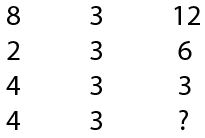# SSC CGL 2014 Tier 1 20 July First Sitting

Instructions

For the following questions answer them individually

Question 181

# Select the correct combination of mathematical signs to replace * signs and to balance the given equation 33*11*3*6 = 115

Instructions

In each of the following questions, find the missing number from the given responses.

Question 182

Question 183

Question 184

#Instructions

For the following questions answer them individually

Question 185

Question 186

# ‘A’ walks 10 metres in front and 10 metres to the right. And after walking staright takes a right turn and walks 5 metres. How far is he now from his starting point?

Instructions

In each of the following questions, one/two statements are given followed by two conclusions/assumptions, I and II. You have to consider the statements to be true, even if they seem to be at variance from commonly known facts. You are to decide which of the given conclusions/assumptions can definitely be drawn from the given statements. Indicate your answer.

Question 187

Question 188

# Statement :Regular reading of newspapers enhances one’s general knowledge.AssumptionsI. Newspaper contains a lot of general knowledge.II. Enhancement of general knowledge enables success in life.

Instructions

For the following questions answer them individually

Question 189

Question 190